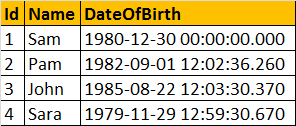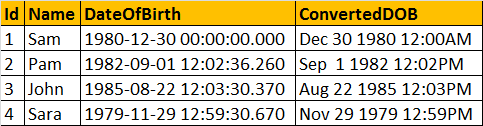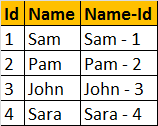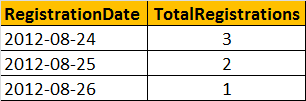Support us .Net Basics C# SQL ASP.NET Aarvi MVC Slides C# Programs Subscribe Download

### Cast and Convert functions in SQL Server - Part 28

To convert one data type to another, CAST and CONVERT functions can be used.

Syntax of CAST and CONVERT functions from MSDN:
CAST ( expression AS data_type [ ( length ) ] )
CONVERT ( data_type [ ( length ) ] , expression [ , style ] )

From the syntax, it is clear that CONVERT() function has an optional style parameter, where as CAST() function lacks this capability.

Consider the Employees Table belowThe following 2 queries convert, DateOfBirth's DateTime datatype to NVARCHAR. The first query uses the CAST() function, and the second one uses CONVERT() function. The output is exactly the same for both the queries as shown below.
Select Id, Name, DateOfBirth, CAST(DateofBirth as nvarchar) as ConvertedDOB
from tblEmployees

Select Id, Name, DateOfBirth, Convert(nvarchar, DateOfBirth) as ConvertedDOB
from tblEmployees

Output:Now, let's use the style parameter of the CONVERT() function, to format the Date as we would like it. In the query below, we are using 103 as the argument for style parameter, which formats the date as dd/mm/yyyy.
Select Id, Name, DateOfBirth, Convert(nvarchar, DateOfBirth, 103) as ConvertedDOB
from tblEmployees

Output:The following table lists a few of the common DateTime styles:For complete list of all the Date and Time Styles, please check MSDN.

To get just the date part, from DateTime
SELECT CONVERT(VARCHAR(10),GETDATE(),101)

In SQL Server 2008, Date datatype is introduced, so you can also use
SELECT CAST(GETDATE() as DATE)
SELECT CONVERT(DATE, GETDATE())

Note: To control the formatting of the Date part, DateTime has to be converted to NVARCHAR using the styles provided. When converting to DATE data type, the CONVERT() function will ignore the style parameter.

Now, let's write a query which produces the following output:In this query, we are using CAST() function, to convert Id (intto nvarchar, so it can be appended with the NAME column. If you remove the CAST() function, you will get an error stating - 'Conversion failed when converting the nvarchar value 'Sam - ' to data type int.'
Select Id, Name, Name + ' - ' + CAST(Id AS NVARCHAR) AS [Name-Id]
FROM tblEmployees

Now let's look at a practical example of using CAST function. Consider the registrations table below.Write a query which returns the total number of registrations by dayQuery:
Select CAST(RegisteredDate as DATE) as RegistrationDate,
COUNT(Id) as TotalRegistrations
From tblRegistrations
Group By CAST(RegisteredDate as DATE)

The following are the differences between the 2 functions.
1. Cast is based on ANSI standard and Convert is specific to SQL Server. So, if portability is a concern and if you want to use the script with other database applications, use Cast().
2. Convert provides more flexibility than Cast. For example, it's possible to control how you want DateTime datatypes to be converted using styles with convert function.

The general guideline is to use CAST(), unless you want to take advantage of the style functionality in CONVERT().

1.Write a query which returns the total number of registrations by Year?
select cast(datepart(year,doj)as int)as 'DOJ',count(id) from tblDate
group by cast(datepart(year,doj) as int)

2.Can anyone advise how to show my graph in chronological by month.
Below is my query

select CAST(DATENAME(Month, '2006/' + Convert(varchar(2), datepart(mm,WP_DATE_ISSUE)) + '/1') AS varchar(15)), count(*) as "Total",
COUNT(CASE WHEN SpecificLocation = 'Level 1' THEN 1
ELSE NULL
END) AS "Pending for Level 1",
COUNT(CASE WHEN SpecificLocation = 'Level 2' THEN 1
ELSE NULL
END) AS "Pending for Level 2",
COUNT(CASE WHEN SpecificLocation = 'Level 3' THEN 1
ELSE NULL
END) AS "Pending for Level 3",
COUNT(CASE WHEN SpecificLocation = 'Approved' THEN 1
ELSE NULL
END) AS "Approved",
COUNT(CASE WHEN SpecificLocation = 'Rejected' THEN 1
ELSE NULL
END) AS "Rejected"
from FWPERMIT
group by CAST(DATENAME(mm, '2006/' + Convert(varchar(2), datepart(mm,WP_DATE_ISSUE)) + '/1') AS varchar(15))

3.4.